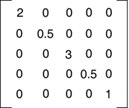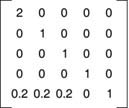# Color Transformation and the Color Matrix

So far we have seen the transformation of graphics shapes from one state to another, but have you ever thought about transforming colors? Why would you want to transform an image's colors? Suppose you wanted to provide grayscale effects, or needed to adjust the contrast, brightness, or even "redness" of an image. For example, images retrieved from video and still cameras often need correction. In these cases, a color matrix is very useful.

As we discussed in earlier chapters, the color of each pixel of a GDI+ image or bitmap is represented by a 32-bit number, of which 8 bits each are used for the red, green, blue, and alpha components. Each of the four components is a number from 0 to 255. For red, green, and blue, 0 represents no intensity and 255 represents full intensity. For the alpha component, 0 represents transparent and 255 represents fully opaque. A color vector includes four items: A, R, G, and B. The minimum values for this vector are (0, 0, 0, 0), and the maximum values are (255, 255, 255, 255).

GDI+ allows the use of values between 0 and 1, where 0 represents the minimum intensity and 1 the maximum intensity. These values are used in a color matrix to represent the intensity and opacity of color components. For example, the color vector with minimum values is (0, 0, 0, 0), and the color vector with maximum values is (1, 1, 1, 1).

In a color transformation we can apply a color matrix on a color vector by multiplying a 4x4 matrix. However, a 4x4 matrix supports only linear transformations such as rotation and scaling. To perform nonlinear transformations such as translation, we must use a 5x5 matrix. The element of the fifth row and the fifth column of the matrix must be 1, and all of the other entries in the five columns must be 0.

The elements of the matrix are identified according to a zero-based index. The first element of the matrix is M, and the last element is M. A 5x5 identity matrix is shown in Figure 10.21. In this matrix the elements M, M, M, and M represent the red, blue, green, and alpha factors, respectively. The element M means nothing, and it must always be 1.

Figure 10.21. An identity matrixNow if we want to double the intensity of the red component of a color, we simply set M equal to 2. For example, the matrix shown in Figure 10.22 doubles the intensity of the red component, decreases the intensity of the green component by half, triples the intensity of the blue component, and decreases the opacity of the color by half (making it semitransparent).

Figure 10.22. A matrix whose components have different intensitiesIn the matrix shown in Figure 10.22, we multiplied the intensity values. We can also add intensity values by using other matrix elements. For example, the matrix shown in Figure 10.23 will double the intensity of the red component and add 0.2 to each of the red, green, and blue component intensities.

Figure 10.23. A color matrix with multiplication and addition10.7.1 The ColorMatrix Class

In this section we will discuss the ColorMatrix class. As you might guess from its name, this class defines a matrix of colors. In the preceding sections we discussed the Matrix class. The ColorMatrix class is not very different from the Matrix class. Whereas the Matrix class is used in general transformation to transform graphics shapes and images, the ColorMatrix class is specifically designed to transform colors. Before we see practical use of the color transformation, we will discuss the ColorMatrix class, its properties, and its methods.

The ColorMatrix class constructor takes an array that contains the values of matrix items. The Item property of this class represents a cell of the matrix and can be used to get and set cell values. Besides the Item property, the ColorMatrix class provides 25 MatrixXY properties, which represent items of the matrix at row (x + 1) and column (y + 1). MatrixXY properties can be used to get and set an item's value.

Listing 10.17 creates a ColorMatrix object with item (4, 4) set to 0.5 (half opacity). Then it sets the values of item (3, 4) to 0.8 and item (1, 1) to 0.3.

Listing 10.17 Creating a ColorMatrix object

```float[][] ptsArray ={
new float[] {1, 0, 0, 0, 0},
new float[] {0, 1, 0, 0, 0},
new float[] {0, 0, 1, 0, 0},
new float[] {0, 0, 0, 0.5f, 0},
new float[] {0, 0, 0, 0, 1}};
ColorMatrix clrMatrix = new ColorMatrix(ptsArray);
if( clrMatrix.Matrix34 <= 0.5)
{
clrMatrix.Matrix34 = 0.8f;
clrMatrix.Matrix11 = 0.3f;
}
```

Section 10.8 will describe how to apply color matrices to the transformation of colors.GDI+ Programming with C#
ISBN: 073561265X
EAN: N/A
Year: 2003
Pages: 145